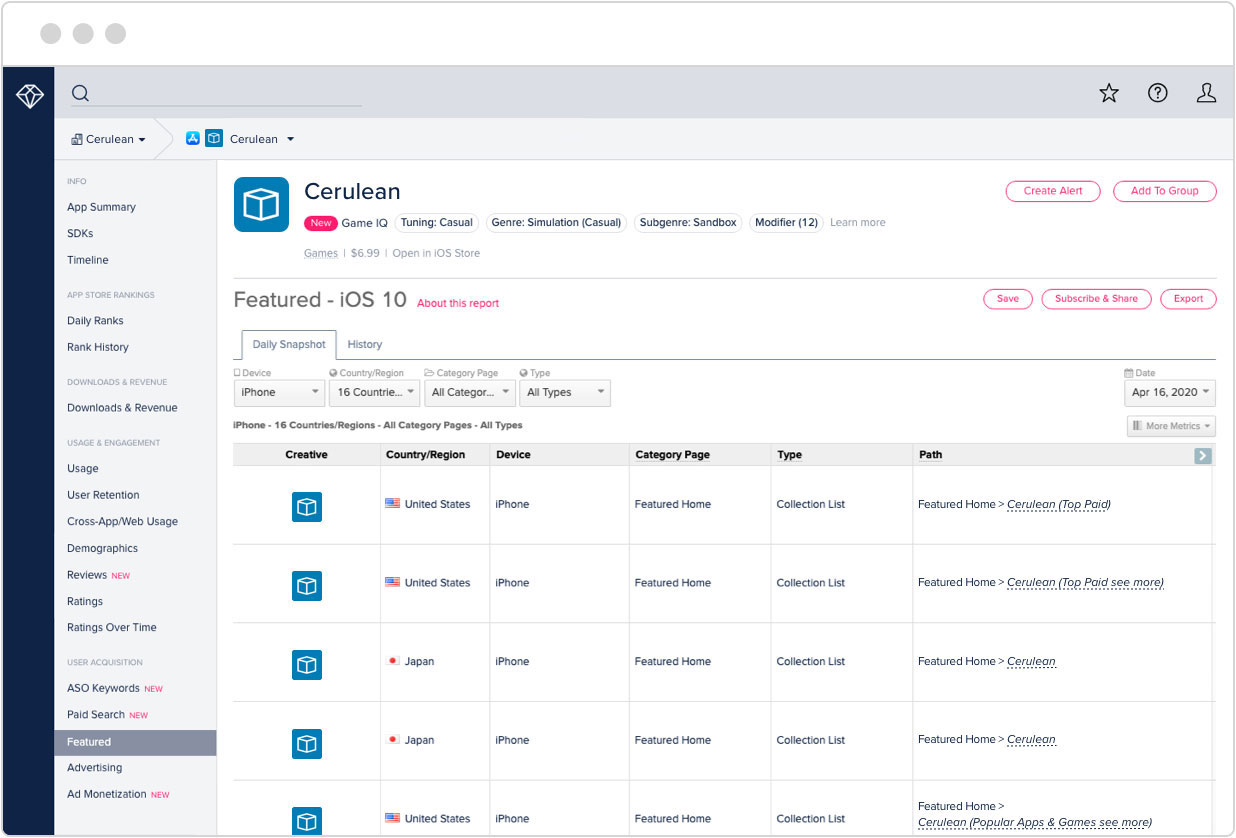# Math Bingo K-6

### 免费注册来发掘更多！### 应用评价和评级### 应用说明

Math Bingo is a two player game that provides practice in addition, subtraction, multiplication, division and other math skills. Twenty different levels of problems can be selected. The levels are keyed to the Common Core Standards and are identified by identification numbers from the standards as well as descriptions. Within each of the levels problems are randomly generated so every game is a unique experience.

Players begin by sitting opposite each other with the iPad between them. The first step is to use a slider to select a marker for each game board. Once both players tap the "GO!" button, the game begins. A problem is displayed on the screen and the players mark answers on the game boards. The first player to get five in a row wins the round.

This app is appropriate for elementary age students and can also be used with middle school students who need more practice to improve math skills.

1
Operations and Algebraic Thinking Add within 20 Integers from 1 to 10 1.OA.6
2
Operations and Algebraic Thinking Add within 20. Integers from 5 to 20 1.OA.6
3
Operations and Algebraic Thinking Add within 20. Integers from 10 to 20 1.OA.6
4
Operations and Algebraic Thinking Add within 20. Integers from 1 to 20 1.OA.6
5
Operations and Algebraic Thinking Subtract within 20. Integers from 1 to 10 1.OA.6
6
Operations and Algebraic Thinking Subtract within 20. Integers from 1 to 20 1.OA.6
7
Operations and Algebraic Thinking Determine the unknown whole number in an addition equation. Integers from 1 to 20 1.OA.8
8
Numbers and Operations in Base Ten Add within 100, including adding a two-digit number and a one-digit number. Integers from 1 to 100 and 1 to 10 1.NBT.4
9
Numbers and Operations in Base Ten Given a two digit number mentally find 10 more or 10 less. Integers from 10 to 100 and 10 1.NBT.5
10
Numbers and Operations in Base Ten Subtract multiples of 10 in the range 10-90 from multiples of 10 in the range 10-90 (positive or zero differences) Integers from 10 to 90 1.NBT.6
11
Operations and Algebraic Thinking Fluently add and subtract within 20 using mental strategies. Integers from 0 to 20 2.OA.2
12
Numbers and Operations in Base Ten Fluently add and subtract within 100 using strategies based on place value, properties of operations, and/or the relationship between addition and subtraction. Integers from 0 to 100 2.NBT.5
13
Numbers and Operations in Base Ten Add up to four two-digit numbers using strategies based on place value and properties of operations. Number Range: Integers from 0 to 1000 2.NBT.6
14
Numbers and Operations in Base Ten Mentally add 10 or 100 to a given number 100–900, and mentally subtract 10 or 100 from a given number 100–900. Number Range: Integers from 10 to 100 and 100 to 900 2.NBT.8
15
Operations and Algebraic Thinking Fluently multiply within 100. Factors from 0 to 10 3.OA.7
16
Operations and Algebraic Thinking Fluently divide within 100. Divisors and Quotients from 0 to 10 3.OA.7
17
Number and Operations in Base Ten Recognize that in a multi-digit whole number, a digit in one place represents ten times what it represents in the place to its right. Dividends from 10 to 1000 and Divisors of 10 or 100 4.NBT.A.1
18
Operations and Algebraic Thinking Use parentheses, brackets, or braces in numerical expressions, and evaluate expressions with these symbols. Integers 1 to 10 5.OA.A.1
19
Expressions and Equations Evaluate expressions at specific values of their variables. Integers 1 to 10 6.EE.A.2c
20
Expressions and Equations Solve real-world and mathematical problems by writing and solving equations of the form x + p = q and px = q for cases in which p, q and x are all nonnegative rational numbers. Integers 1 to 10 6.EE.B.7